# CBSE Question Paper 2007 class 12 Chemistry## myCBSEguide App

CBSE, NCERT, JEE Main, NEET-UG, NDA, Exam Papers, Question Bank, NCERT Solutions, Exemplars, Revision Notes, Free Videos, MCQ Tests & more.

CBSE Question Paper 2007 class 12 Chemistry conducted by Central Board of Secondary Education, New Delhi in the month of March 2007. CBSE previous year question papers with the solution are available in the myCBSEguide mobile app and website. The Best CBSE App for students and teachers is myCBSEguide which provides complete study material and practice papers to CBSE schools in India and abroad.

CBSE Question Paper 2007 class 12 Chemistry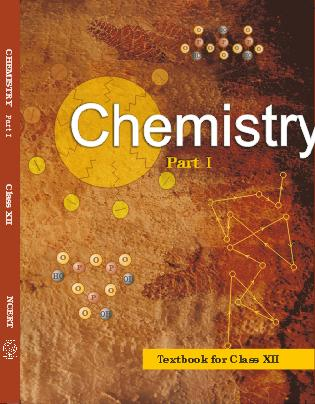## Class 12 Chemistry list of chapters

1. The Solid State
2. Solutions
3. Electrochemistry
4. Chemical Kinetics
5. Surface Chemistry
6. General Principles and Processes of Isolation of Elements
7. The p-Block Elements
8. The d and f Block Elements
9. Coordination Compounds
10. Haloalkanes and Haloarenes
11. Alcohols, Phenols and Ethers
12. Aldehydes, Ketones and Carboxylic Acids
13. Amines
14. Biomolecules
15. Polymers
16. Chemistry in Everyday life

## CBSE Question Paper 2007 class 12 Chemistry

### General Instructions

1. All questions are compulsory.
2. Marks for each question are indicated against it.
3. Question numbers 1 to 5 are very short-answer questions, carrying 1 mark each. Answer these in one word or about one sentence each.
4. Question numbers 6 to 12 are short-answer questions, carrying 2 marks each. Answer these in about 30 words each.
5. Question numbers 13 to 24 are short-answer questions of 3 marks each. Answer these in about 40 words each.
6. Question numbers 25 to 27 are long-answer questions of 5 marks each. Answer these in about 70 words each.
7. Use Log Tables, if necessary Use of calculators is not permitted.

1. What is the coordination number in a rock salt type structure? 

2. State Raoult’s law for a binary solution containing volatile components. 

3. What is meant by order of a reaction being zero? 

4. Write the IUPAC name of the following compound: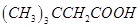5. Mention one commercial use of N,N-Dimethylaniline (DMA). 

6. State as a mathematical formula the de Broglie relationship for moving particles. What experimental evidence is available for this concept? 

OR

Specify the ranges of values for quantum numbers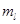and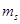for an electron in an atom when the n quantum number value for it is 2. What is the significance of these values for the orbitals? 

7. When can an endothermic process be spontaneous? Give an example of such a process. 

8. Write balanced chemical equations for the following reactions: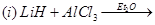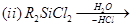9. Explain any one of the following statements: 

(i) The transition metals are well known for the formation of interstitial compounds.

(ii) The largest number of oxidation states are exhibited by manganese in the first series of transition elements.

10. Draw the three-dimensional representations of (R)- and (S)- butan-2-ol. 

11. Write chemical reaction equations to illustrate the following reactions: 

(i) Williamson synthesis of ethers

(ii) Reimer-Tiemann reaction

12. Distinguish between addition polymers and condensation polymers and give one example of each class. 

13. Answer the following in the light of MO theory: 

(a) Which has a higher bond order,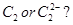(b) Which species is not likely to exist,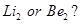OR

(a) Compare the structural shapes of the following species: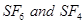(b) What type of intermolecular forces exist between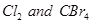present in a mutual solution? 

14. (a) Name an element with which silicon can be doped to give an n-type semiconductor.

(b) Which type of crystals exhibits piezoelectricity? 

15. The vapour pressure of water is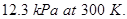Calculate the vapour pressure of a one molal solution of a non-volatile non-ionic solute in water. 

16. Using the values of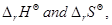given herein, calculate the standard molar Gibbs energy of formation,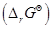for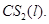Given: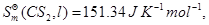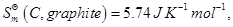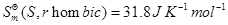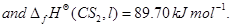17. The rates of most reactions double when their temperature is raised from 298 K to 308 K. Calculate activation energy of such a reaction.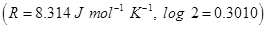18. State what is observed when

(i) the electrodes connected to a battery are dipped into a sol.

(ii) an electrolyte solution is added to a sol.

(iii) an emulsion is subjected to high speed centrifugation. 

19. Answer the following questions: 

(i) Which element in the first series of transition elements does not exhibit variable oxidation states and why?

(ii) What happens when a solution of copper (II) sulphate is saturated with ammonia?

(iii) Why do actinoids, in general, exhibit a greater range of oxidation states than the lanthanoids?

20. (a) Illustrate the following with an example each:

(ii) Coordination isomerism

(b) Why is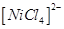paramagnetic?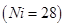21. Write the nuclear reactions for the following radioactive changes: 

(i)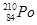undergoes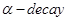(ii)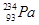undergoes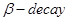(iii)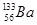undergoes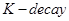(You can put ‘X’ for the symbol which is not correctly known)

22. Explain the mechanism of nucleophilic addition to a carbonyl group and give one example of such addition reactions. 

23. Write the chemical equations for the following chemical reactions: 

(a) 1-Nitropropene is prepared from acetaldehyde

(b) Benzonitrile is converted to acetophenone

(c) A primary amine is prepared from a primary alkyl halide

24. Mention one important use of each of the following: 

(i) Equanil

(ii) Sucralose

(iii) Carbon fibres

25. (a) Write the formulation for the galvanic cell in which the reaction,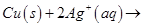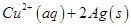takes place.

Identify the cathode and the anode reactions in it.

(b) Write Nernst equation and calculate the emf of the following cell: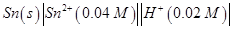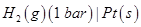(Given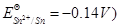[2, 3]

OR

(a) Explain with one example each the terms weak and strong electrolytes.

(b) Write the Nernst equation and calculate the emf of the following cell: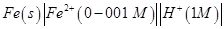(Given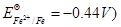[2, 3]

26. (a) How would you account for any two of the following:

(i)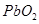is a stronger oxidising agent than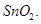(ii)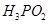acts as a monobasic acid.

(iii) The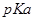value for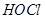is higher than that of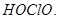(b) Draw the structures of the following species:

(i) Peroxodisulphuric acid,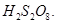(ii) Xenon tetrafluoride,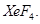[2, 3]

OR

(a) Assign reasons for any two of the following observations:

(i) The lower oxidation state becomes more stable with increasing atomic number in Group 13.

(ii) Hydrogen iodide is a stronger acid than hydrogen fluoride in aqueous solution.

(iii) The basic character among the hydrides of Group 15 elements decreases with increasing atomic numbers.

(b) Draw the structural formula for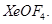[2, 3]

27. (a) Name the three major classes of carbohydrates and give the distinctive characteristic of each class.

(b) What are nucleotides? Name two classes of nitrogen containing bases found amongst nucleotides. [3, 2]

OR

(a) Describe the classification of lipids based on their chemical compositions. Mention the chief chemical characteristic of each class.

(b) Explain the term ‘mutarotation’. [3, 2]

These are questions only. To view and download complete question paper with solution install myCBSEguide App from google play store or login to our student dashboard.

## Chemistry Question Paper 2007

Download class 12 Chemistry question paper with solution from best CBSE App the myCBSEguide. CBSE class 12 Chemistry question paper 2007 in PDF format with solution will help you to understand the latest question paper pattern and marking scheme of the CBSE board examination. You will get to know the difficulty level of the question paper. CBSE question papers 2007 for class 12 Chemistry have 27 questions with solution.

## Previous Year Question Paper for class 12 2017 Free PDF Download

CBSE question papers 2018, 2017, 2016, 2015, 2014, 2013, 2012, 2011, 2010, 209, 2008, 2007, 2006, 2005 and so on for all the subjects are available under this download link. Practicing real question paper certainly helps students to get confidence and improve performance in weak areas.

To download CBSE Question Paper 2007 class 12 Accountancy, Chemistry, Physics, History, Political Science, Economics, Geography, Computer Science, Home Science, Accountancy, Business Studies and Home Science; do check myCBSEguide app or website. myCBSEguide provides sample papers with solution, test papers for chapter-wise practice, NCERT solutions, NCERT Exemplar solutions, quick revision notes for ready reference, CBSE guess papers and CBSE important question papers. Sample Paper all are made available through the best app for CBSE students and myCBSEguide website.